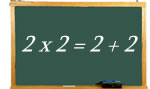Logic puzzles, riddles, math puzzles and brainteasers - pzzls.com

Vandaag is het 7 June 2023

Sort - Oldest on top
Page: << 4 5 6 7 8 >>

# Letter folding - math puzzle

Difficulty:Rating: 2.6/5.0

If you would fold a letter in two fifty times, how thick should the enveloppe be that can fit the letter? Assume that the thickness of a piece of paper is about 0.05 mm.

# 2 x 2 = 2 + 2 - math puzzle

Difficulty:Rating: 2.9/5.0

 Two times two is equal to two and two. But are there more number pairs for which the sum of the numbers is is equal to the product of the numbers? The numbers may be different.# Chess-board - math puzzle

Difficulty:Rating: 3.0/5.0

 How many different squares are located on a chess-board?